# Federal Department of Home Affairs FDHA Federal Office

• Slides: 24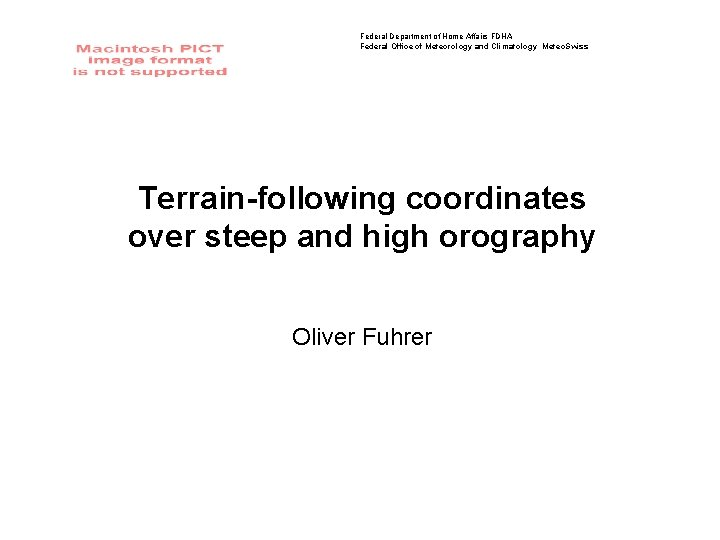Federal Department of Home Affairs FDHA Federal Office of Meteorology and Climatology Meteo. Swiss Terrain-following coordinates over steep and high orography Oliver FuhrerOutline • Terrain-following coordinates • Two idealized tests • Atmosphere at rest • Constant advection • Possible remedy: SLEVE • How to specify SLEVE parameters?Overview • Terrain-following coordinates have many advantages. . . - rectangular computational mesh - lower boundary condition - stretching → boundary layer representation • But also many disadvantages. . . - additional terms - horizontal pressure gradient formulation (Janjic, 1989) - metric terms (Klemp et al. , 2003) - truncation error (Schär et al. , 2002)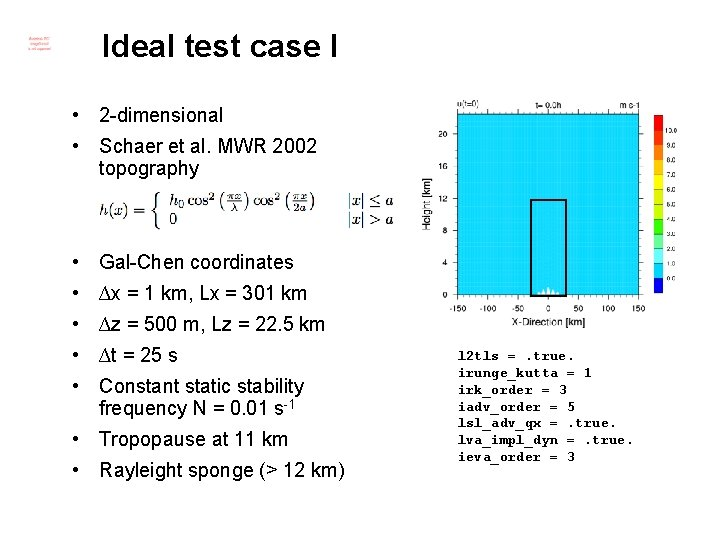Ideal test case I • 2 -dimensional • Schaer et al. MWR 2002 topography • Gal-Chen coordinates • ∆x = 1 km, Lx = 301 km • ∆z = 500 m, Lz = 22. 5 km • ∆t = 25 s • Constant static stability frequency N = 0. 01 s-1 • Tropopause at 11 km • Rayleight sponge (> 12 km) l 2 tls =. true. irunge_kutta = 1 irk_order = 3 iadv_order = 5 lsl_adv_qx =. true. lva_impl_dyn =. true. ieva_order = 3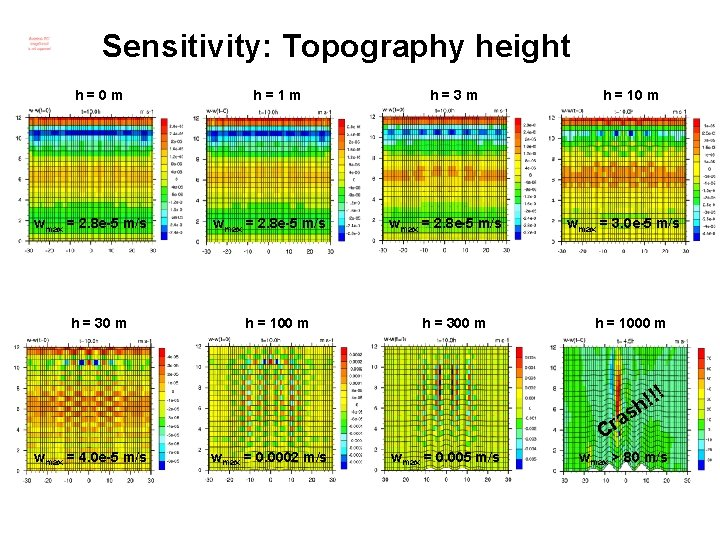Sensitivity: Topography height h=0 m wmax = 2. 8 e-5 m/s h = 30 m h=1 m wmax = 2. 8 e-5 m/s h = 100 m h=3 m wmax = 2. 8 e-5 m/s h = 300 m h = 10 m wmax = 3. 0 e-5 m/s h = 1000 m !! ! h as r C wmax = 4. 0 e-5 m/s wmax = 0. 0002 m/s wmax = 0. 005 m/s wmax > 80 m/sSensitivity: Overview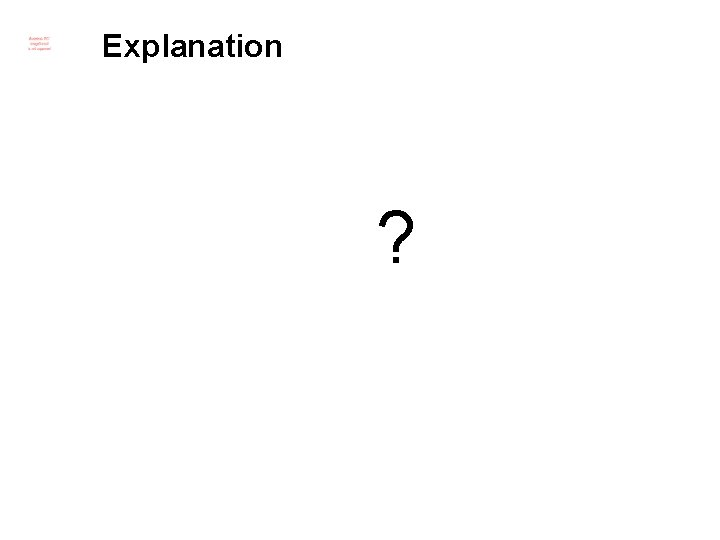Explanation ?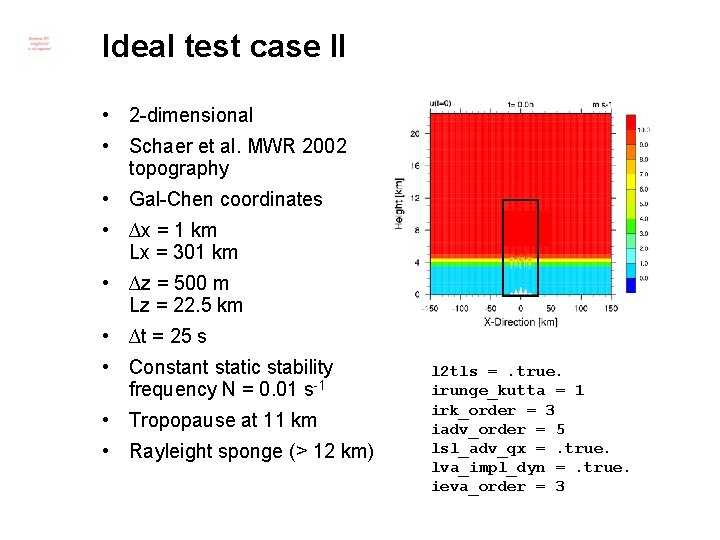Ideal test case II • 2 -dimensional • Schaer et al. MWR 2002 topography • Gal-Chen coordinates • ∆x = 1 km Lx = 301 km • ∆z = 500 m Lz = 22. 5 km • ∆t = 25 s • Constant static stability frequency N = 0. 01 s-1 • Tropopause at 11 km • Rayleight sponge (> 12 km) l 2 tls =. true. irunge_kutta = 1 irk_order = 3 iadv_order = 5 lsl_adv_qx =. true. lva_impl_dyn =. true. ieva_order = 3Sensitivity: Topography height h=0 m wmax = 0. 0001 m/s h = 30 m h=1 m wmax = 0. 001 m/s h = 100 m h=3 m wmax = 0. 002 m/s h = 300 m h = 10 m wmax = 0. 007 m/s h = 1000 m !! ! h as r C wmax = 0. 02 m/s wmax = 0. 07 m/s wmax = 0. 4 m/s wmax > 80 m/s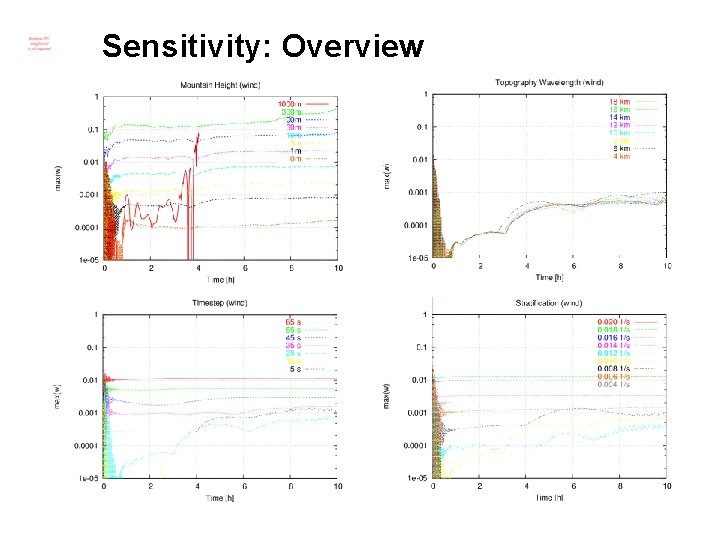Sensitivity: OverviewExplanation • Advection of intensive quantity • Theoretical analysis of trunction error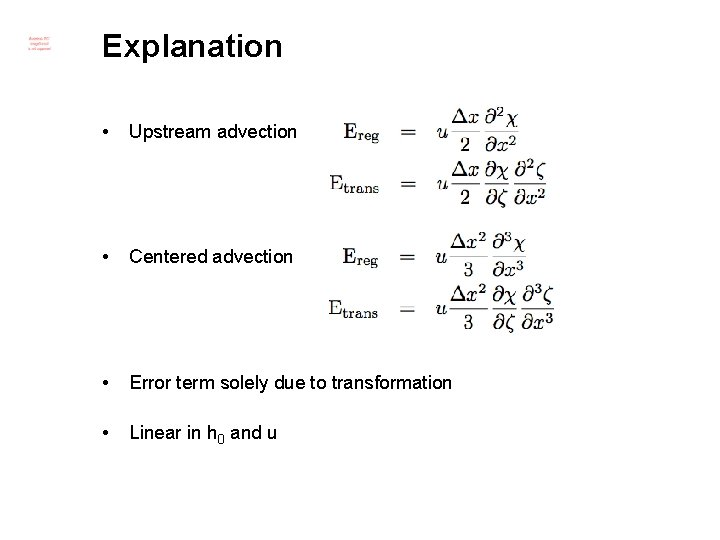Explanation • Upstream advection • Centered advection • Error term solely due to transformation • Linear in h 0 and uSolution Gal-Chen SLEVE 2 zmin = 13. 3 m zmin = 4. 3 m zmin = 16. 5 m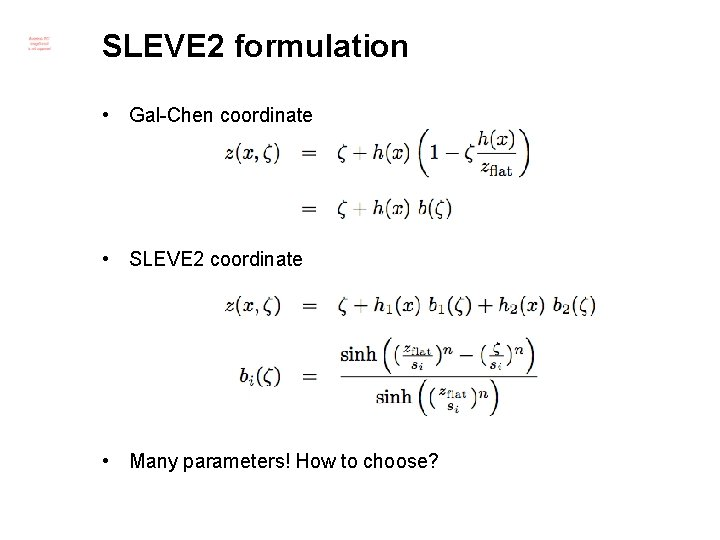SLEVE 2 formulation • Gal-Chen coordinate • SLEVE 2 coordinate • Many parameters! How to choose?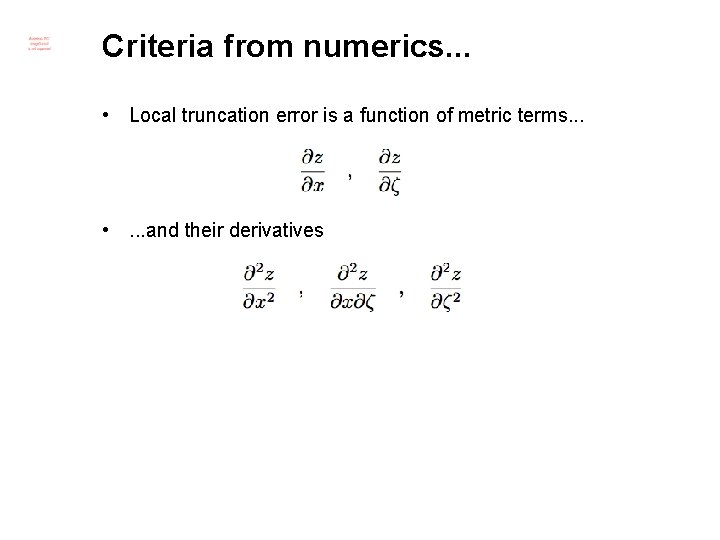Criteria from numerics. . . • Local truncation error is a function of metric terms. . . • . . . and their derivativesGal-Chen gridSLEVE 2 gridOptimal grid? • Optimize cost function • We have neglected cross-derivatives • Using n=2 for simplicity • Choose weights i inspired from SLEVE 2 values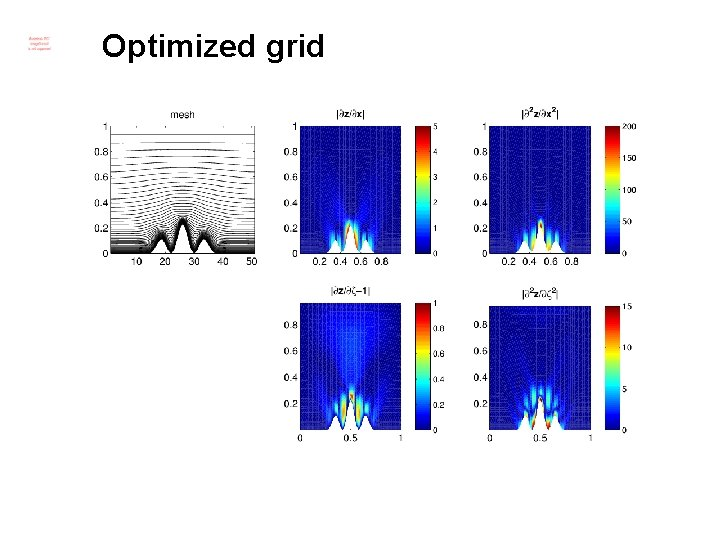Optimized grid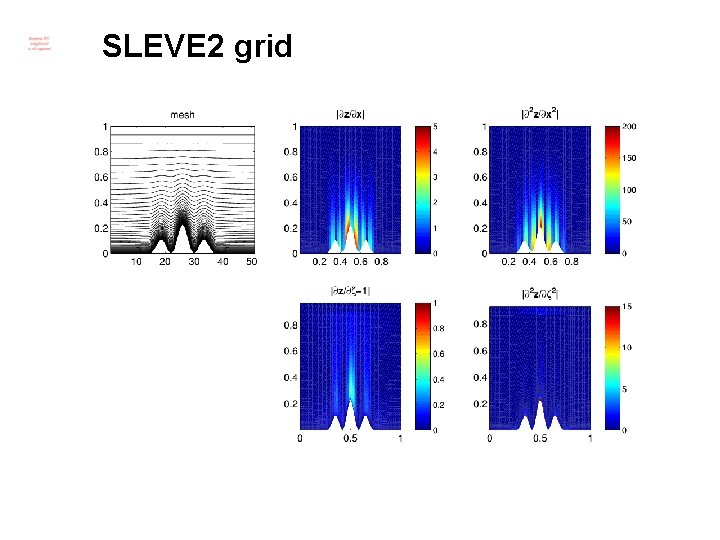SLEVE 2 grid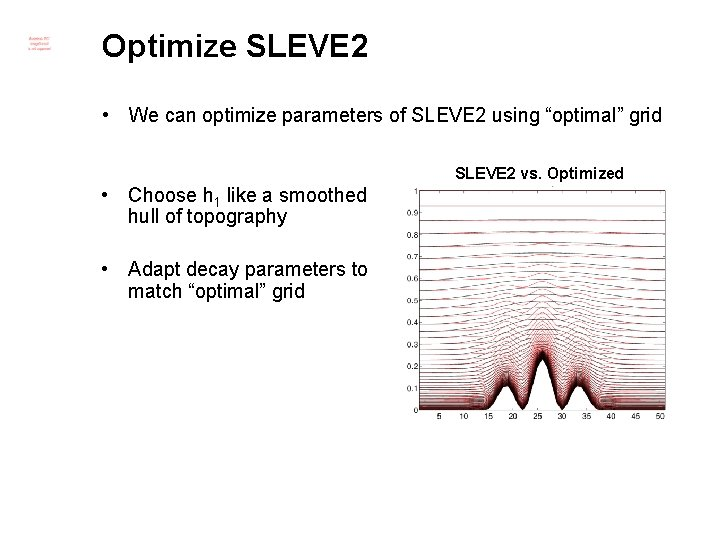Optimize SLEVE 2 • We can optimize parameters of SLEVE 2 using “optimal” grid SLEVE 2 vs. Optimized • Choose h 1 like a smoothed hull of topography • Adapt decay parameters to match “optimal” gridOptimize SLEVE 2 • We can optimize parameters of SLEVE 2 using “optimal” grid Optimized SLEVE 2 vs. OptimizedConclusions • COSMO shows considerable truncation errors in presence of steep and high topography • Both for atmosphere at rest and constant advection test cases • Better vertical coordinate transformations (SLEVE 2) may reduce the amplitude at higher levels • But they have many parameters to choose and effects near the landsurface can be critical • Grid-optimization with numerically motivated cost function. . . • may be a tool to choose parameters intelligently • may assist in formulation of new vertical coordinates • also relies on some tuning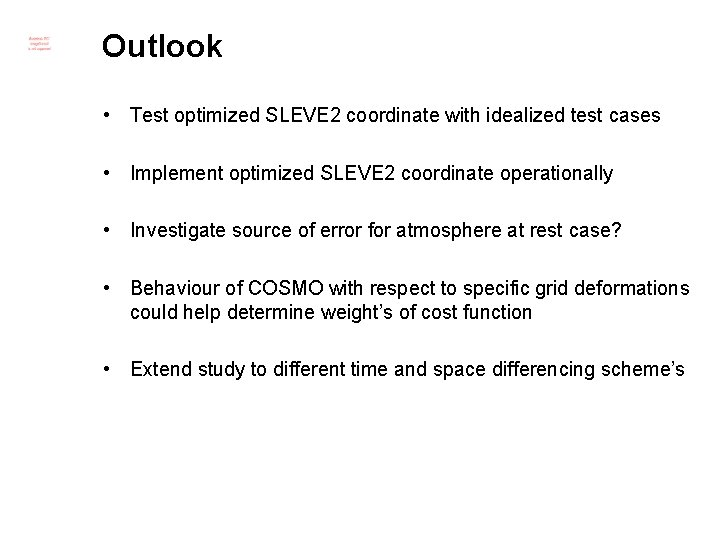Outlook • Test optimized SLEVE 2 coordinate with idealized test cases • Implement optimized SLEVE 2 coordinate operationally • Investigate source of error for atmosphere at rest case? • Behaviour of COSMO with respect to specific grid deformations could help determine weight’s of cost function • Extend study to different time and space differencing scheme’s